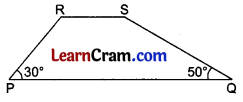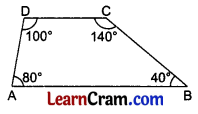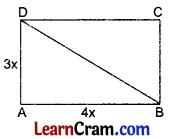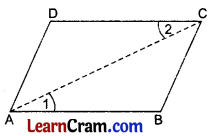# DAV Class 8 Maths Chapter 11 Worksheet 2 Solutions

The DAV Maths Class 8 Solutions and DAV Class 8 Maths Chapter 11 Worksheet 2 Solutions of Understanding Quadrilaterals offer comprehensive answers to textbook questions.

## DAV Class 8 Maths Ch 11 WS 2 Solutions

Question 1.
PQRS is a trapezium with PQ || SR. If ∠P = 30°, ∠Q = 50°, find ∠R and ∠S.
Solution:In trapezium PQRS
PQ || SR
∴ ∠P + ∠S = 180° [Sum of interior angles]
30° + ∠S = 180°
∴ ∠S = 180° – 30° = 150°
Similarly ∠Q + ∠R = 180°
50° + ∠R = 180°
∴ ∠R = 180° – 50° = 130°.Question 2.
ABCD is a quadrilateral with ∠A = 80°, ∠B = 40°, ∠C = 140°, ∠D = 100°.
(i) Is ABCD a trapezium?
(ii) Is ABCD a parallelogram?
Solution:(i) ∠A + ∠D = 80° +100° = 180°
and ∠B + ∠C = 40° + 140° = 180°
The sum of the interior angles is 180°
∴ AB || DC
Hence it is a trapezium.

(ii) ∠A + ∠B = 80° + 40° = 120°.
The sum of the interior angle is not 180°.
∴ AD is not parallel to BC.
Hence it is not a parallelogram.

Question 3.
One of the angles of a parallelogram is 75°. Find the measures of the of the remaining angles parallelogram.
Solution:ABCD is a parallelogram in which ∠A = 75°
∴ ∠A = ∠C [Opposite angles of the parallelogram]
∴ ∠C = 75°
∠A + ∠B = 180° [Adjacent angles]
75° + ∠B = 180°
∴ ∠B = 180° – 75° = 105°
∠B = ∠D [Opposite angles of the parallelogram]
105° = ∠D
∴ ∠D = 105°.Question 4.
Two adjacent angles of a parallelogram are in the ratio 1 : 5. Find all the angles of the parallelogram.
Solution:
Let the two adjacent angles be x° and 5x°.
∴ x° + 5x° = 180° [Sum of adjacent angles]
⇒ 6x = 180
⇒ x = 30
Hence the required adjacent angles are 1 × 30 = 30° and 5 × 30 = 150°.

Question 5.
The exterior angle of a parallelogram is 110°. Find the angles of the parallelogram.
Solution:∠A + ∠DAE = 180° [Linear pairs]
∠A + 110° = 180°
∴ ∠A = 180° – 110° = 70°
∠C = ∠A = 70° [Opposite angles of a || gram]
∠A + ∠B = 180° [Adjacent angles]
70° + ∠B = 180°
∴ ∠B = 180° – 70° = 110°
∠B = ∠D [Opposite angles of the parallelogram]
110° = ∠D
Hence ∠A = 70°, ∠B = 110°, ∠C = 70° and ∠D = 110°.

Question 6.
Two adjacent sides of a parallelogram are in the ratio 3 : 8 and its perimeter is 110 cm. Find the sides of the parallelogram.
Solution:Let AB be 8x cm and AD be 3x cm
∴ Perimeter = 2[AB + AD]
⇒ 110 = 2[8x + 3x]
⇒ 110 = 2 × 11x
⇒ 22x = 110
⇒ x = $$\frac{110}{22}$$ = 5
∴ AB = 8 × 5 = 40 cm
BC = AD = 3 × 5 = 15 cm
DC = AB = 40 cm.Question 7.
One side of a parallelogram is $$\frac{3}{4}$$ times its adjacent side. If the perimeter of the parallelogram is 70 cm, find the sides of the parallelogram.
Solution:
Let one side of the parallelogram be x cm
∴ The adjacent side = $$\frac{3}{4}$$x cm
∴ Perimeter = 2[l + b]
⇒ 70 = 2[x + $$\frac{3}{4}$$x]
⇒ 70 = 2 × $$\frac{7}{4}$$x
⇒ 70 = $$\frac{7}{2}$$x
⇒ x = 70 × $$\frac{2}{7}$$
⇒ x = 20
∴ The required sides are 20 cm = $$\frac{3}{4}$$ × 20 = 15 cm, 20 cm and 15 cm.

Question 8.
ABCD is a parallelogram whose diagonals intersect each other at right angles. If the length of the diagonals is 8 cm and 8 cm, find the lengths of all the sides of the parallelogram.
Solution:AC = 8 cm
∴ AO = 4 cm
BD = 6 cm
∴ BO = 3 cm
[The diagonals of a parallelogram bisect each other]
∠AOB = 90° [Given]
∴ In rt. ∆AOB
AB2 = AO2 + BO2 [From Pythagoras Theorem]
⇒ AB2 = (4)2 + (3)2
⇒ AB2 = 16 + 9
⇒ AB2 = 25
⇒ AB = 5 cm
∴ ABCD is a rhombus.
[∵ If the diagonals of a quadrilateral bisect each other at 90° then it is a rhombus]
Hence AB = BC = CD = DA = 5 cm.

Question 9.
In the given figure, one pair of adjacent sides of a parallelogram is in the ratio 3 : 4. If one of its angles, ∠A is a right angle and diagonal $$\overline{\mathrm{BD}}$$ = 10 cm, find the
(i) lengths of the sides of the parallelogram
(ii) perimeter of the parallelogram.
Solution:∠A = 90° [Given]
In rt. ∆ABD,
BD2 = AB2 + AD2 [From Pythagoras Theorem]
⇒ (10)2 = (4x)2 + (3x)2
⇒ 100 = 16x2 + 9x2
⇒ 100 = 25x2
⇒ x2 = $$\frac{100}{25}$$ = 4
∴ x = 2
Hence AB = 4 × 2 = 8 cm
AD = 3 × 2 = 6 cm
BC = AD = 6 cm
CD = AB = 8 cmQuestion 10.
ABCD is a quadrilateral in which $$\overline{\mathrm{AB}}=\overline{\mathrm{CD}}$$ and $$\overline{\mathrm{AD}}=\overline{\mathrm{BC}}$$. Show that it is a parallelogram.
[Hint: Draw one of the diagonals]
Solution:Draw diagonal AC
$$\overline{\mathrm{AB}}=\overline{\mathrm{CD}}$$
$$\overline{\mathrm{AD}}=\overline{\mathrm{BC}}$$ [Given]
$$\overline{\mathrm{AC}}=\overline{\mathrm{AC}}$$ [Common]
∴ $$\overline{\mathrm{AB}} \| \overline{\mathrm{DC}}$$
Similarly $$\overline{\mathrm{AD}} \| \overline{\mathrm{BC}}$$